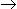# Chemical Engineering - Chemical Reaction Engineering

Exercise : Chemical Reaction Engineering - Section 9
6.
For the gaseous reaction 2AB, where the feed consists of 50 mole % A and 50 mole % inerts, the expansion factor is
1
-0.5
-0.25
0
Explanation:
No answer description is available. Let's discuss.

7.
The molecularity and the order of reaction respectively, for the hydrolysis of methyl acetate in presence of acids are
2 & 1
11 & 2
2 && 2
1 & 1
Explanation:
No answer description is available. Let's discuss.

8.
The irreversible reaction is a special case of reversible reaction, if the
equilibrium constant is 1.
conversion of the reactant at equilibrium condition is zero.
reactant concentration at equilibrium condition is zero.
all (a), (b) and (c).
Explanation:
No answer description is available. Let's discuss.

9.
A pollutant P degrades according to first order kinetics. An aqueous stream containing P at 2 kmole/m3 and volumetric flow rate 1m3 /h requires a mixed flow reactor of volume V to bring down the pollutant level to 0.5 kmole/m3 . The inlet concentration of the pollutant is now doubled and the volumetric flow rate is tripled. If the pollutant level is to be brought down to the same level of 0.5 kmole/m3 , the volume of the mixed flow reactor should be increased by a factor of
7
6
3
7/3
Explanation:
No answer description is available. Let's discuss.

10.
For the liquid phase parallel reactions:
R, rR = K1.CA2; E1 = 80 KJ/mole
S, rs = K1.CA ; E2 = 120 KJ/mole
The desired product is R. A higher selectivity of R will be achieved, if the reaction is conducted at
low temperature in a CSTR.
high temperature in a CSTR.
low temperature in a PFR.
high temperature in a PFR.